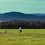# Can $2018^{2}$ be represented as $a^{3}+b^{3}+c^{3}+d^{3}$? Here$a,b,c,d\in Z$

I haven't find a concrete supportive example nor a proof of non-existence of such a representation yet.

But there are still some clues :

……………………………………………………………………………………………………………

First, realize $2018^{2}\equiv 4 (mod9)$,

and $\forall x\in Z,x^{3}\equiv 0,\pm 1(mod9)$ .

So if $2018^{2}$ can be represented as $a^{3}+b^{3}+c^{3}+d^{3}$ for some $a,b,c,d\in Z$,

we certainly get $a^{3}\equiv b^{3}\equiv c^{3}\equiv d^{3}\equiv 1(mod9)$.

And further, $a,b,c,d\in \{9k+1|k\in Z\}\cup\{9k+4|k\in Z\}\cup\{9k+7|k\in Z\}$. ………………………………………………………………………………………………………………

_' Can,or cannot,this is a question! 'Note by Haosen Chen
3 years, 5 months ago

This discussion board is a place to discuss our Daily Challenges and the math and science related to those challenges. Explanations are more than just a solution — they should explain the steps and thinking strategies that you used to obtain the solution. Comments should further the discussion of math and science.

When posting on Brilliant:

• Use the emojis to react to an explanation, whether you're congratulating a job well done , or just really confused .
• Ask specific questions about the challenge or the steps in somebody's explanation. Well-posed questions can add a lot to the discussion, but posting "I don't understand!" doesn't help anyone.
• Try to contribute something new to the discussion, whether it is an extension, generalization or other idea related to the challenge.

MarkdownAppears as
*italics* or _italics_ italics
**bold** or __bold__ bold
- bulleted- list
• bulleted
• list
1. numbered2. list
1. numbered
2. list
Note: you must add a full line of space before and after lists for them to show up correctly
paragraph 1paragraph 2

paragraph 1

paragraph 2

[example link](https://brilliant.org)example link
> This is a quote
This is a quote
    # I indented these lines
# 4 spaces, and now they show
# up as a code block.

print "hello world"
# I indented these lines
# 4 spaces, and now they show
# up as a code block.

print "hello world"
MathAppears as
Remember to wrap math in $$ ... $$ or $ ... $ to ensure proper formatting.
2 \times 3 $2 \times 3$
2^{34} $2^{34}$
a_{i-1} $a_{i-1}$
\frac{2}{3} $\frac{2}{3}$
\sqrt{2} $\sqrt{2}$
\sum_{i=1}^3 $\sum_{i=1}^3$
\sin \theta $\sin \theta$
\boxed{123} $\boxed{123}$

Sort by:

This is still an open question. See (2) and (3) here.

- 3 years, 5 months ago

Thank you,sir Pi Han Goh. My note was inspired by a problem in 2002 IMO proposal

━ Find the smallest positive integer n satisfying : the diophantine equation $x_{1}^{3}+x_{2}^{3}+...+x_{n}^{3}=2002^{2002}$ have integer solution ,

- 3 years, 5 months ago

Ah good to know.

Originally, I spent over an hours trying to come up with a proof for your question that no solution exists via cubic residues but I failed badly so I decided to look it up.

- 3 years, 5 months ago

Oh,I'm sorry,sir. I said I was inspired because $2002^{2002}=(2002^{667})^{3}\cdot (1000^{3}+1000^{3}+1^{3}+1^{3})$ and then I wonder if $2018^{2}$ can be done like $2002^{1}$,since $2018\equiv 2(mod3)$. So …

I'm sorry but you might fail to look it up.

- 3 years, 5 months ago

No, I totally got it. "I looked it up" = I give up because I don't know how to solve it, so I tried the internet for the answers.

- 3 years, 5 months ago